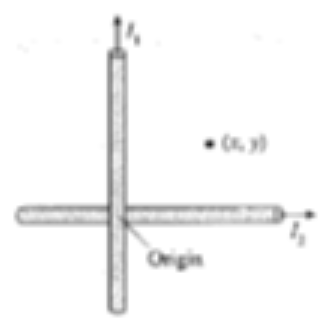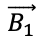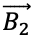# Problem: Suppose you need to find the magnetic field near the intersection of two long, straight, current-carrying wires. Assume that one wire lies directly on top of the other. Let the intersection of the wires be the origin of a coordinate system and let the point of interest, which is in the same plane, have coordinates (x,y). Recall that the magnetic field is a vector, having both a magnitude and a direction.a. What is the direction of magnetic field  due to current I1?. Explainb. Write an expression for the magnitude of .c. What is the direction of magnetic field  due to the current I2? Explain.d. Write an expression for the magnitude of e. What are the only two possible directions for the net magnetic field at this point?f. Would knowing I1 &gt; I2 be enough information to determine the direction of the net magnetic field? Why or why not?g. Let a magnetic field pointing out of the page have a positive value, one pointing into the page a negative value. This is actually B2, the magnetic field along the z-axis. Use your results for parts a-d to write an expression for B2 at position (x,y). This will be a symbolic expression in terms of quantities defined on the figure.

###### FREE Expert Solution

Magnetic field around a wire carrying current:

$\overline{){\mathbf{B}}{\mathbf{=}}\frac{{\mathbf{\mu }}_{\mathbf{0}}\mathbf{i}}{\mathbf{2}\mathbf{\pi }\mathbf{r}}}$

a.

At the point with the dot, the magnetic field due to the wire carrying current I1 is B1.###### Problem Details

Suppose you need to find the magnetic field near the intersection of two long, straight, current-carrying wires. Assume that one wire lies directly on top of the other. Let the intersection of the wires be the origin of a coordinate system and let the point of interest, which is in the same plane, have coordinates (x,y). Recall that the magnetic field is a vector, having both a magnitude and a direction.a. What is the direction of magnetic fielddue to current I1?. Explain

b. Write an expression for the magnitude of.

c. What is the direction of magnetic fielddue to the current I2? Explain.

d. Write an expression for the magnitude ofe. What are the only two possible directions for the net magnetic field at this point?

f. Would knowing I1 > I2 be enough information to determine the direction of the net magnetic field? Why or why not?

g. Let a magnetic field pointing out of the page have a positive value, one pointing into the page a negative value. This is actually B2, the magnetic field along the z-axis. Use your results for parts a-d to write an expression for B2 at position (x,y). This will be a symbolic expression in terms of quantities defined on the figure.